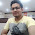### Program to calculate sum,average and division of marks in C++ Language

Introduction: In previous articles i explained the program for Else if ladder or multiple else if to check the day corresponding to number in C++ Language and Else if ladder to perform operation on two numbers based on operator and How to find greatest of three numbers using multiple if statement and Print table of entered number using For loop and How to find month name corresponding to number using switch case.

Description: In this article i have written a program to calculate the total marks of student ,average marks and based on average marks, it calculates the division using else-if ladder or we can say multiple else-if.
On run it will first prompt user to enter name,class,roll number and marks in each subject.Then it will calculate the total marks obtained,average of marks and division achieved by the student.

Implementation: Let's understand by creating the program

#include<conio.h>
#include<iostream.h>
main()
{
clrscr();
char name;
int roll,clas;
float hindi,eng,math,sci,art,comp,total,avg;
cout<<"Enter name of student:= ";
cin>>name;
cout<<"Enter class of student:=";
cin>>clas;
cout<<"Enter rollno of student:=";
cin>>roll;
cout<<"Enter marks in hindi:=";
cin>>hindi;
cout<<"Enter marks in english:=";
cin>>eng;
cout<<"Enter marks in math:=";
cin>>math;
cout<<"Enter marks in science:=";
cin>>sci;
cout<<"Enter marks in art:=";
cin>>art;
cout<<"Enter marks in computers:=";
cin>>comp;
total=hindi+eng+math+sci+art+comp;
avg=total/6;
cout<<"\n************Student's Marks Record*************\n";
cout<<"Student's name:="<<name;
cout<<"\nClass:="<<clas;
cout<<"\nRoll No:="<<roll;
cout<<"\nMarks in Hindi:="<<hindi;
cout<<"\nMarks in English:="<<eng;
cout<<"\nMarks in Math:="<<math;
cout<<"\nMarks in Science:="<<sci;
cout<<"\nMarks in Art="<<art;
cout<<"\nMarks in computer="<<comp;
cout<<"\nTotal Marks:="<<total;
cout<<"\nAverage Marks="<<avg;

if(avg>=80)
{
cout<<"\n Merit";
}
else if(avg>=60)
{
cout<<"\n First Division";
}
else if(avg>=50)
{
cout<<"\n Second Division";
}
else if(avg>=35)
{
cout<<"\n Pass";
}
else
{
cout<<"\n Fail";
}
getch();
}

• Run the program using Ctrl+F9
Output:

Enter name of student:=Karan
Enter class of student:=12
Enter rollno of student:=18
Enter marks in hindi:=77
Enter marks in english:=67
Enter marks in math:=80
Enter marks in science:=75
Enter marks in arts=68
Enter marks in computer:=88

****************Student's Marks Record****************
Student's name:=Karan
Class:=12
Roll No:=18
Marks in Hindi:=77
Marks in English:=67
Marks in Math:=80
Marks in Science:=75
Marks in Art:=68
Marks in Computer:=88
Total Marks:=455
Average Marks:=75.83
First Division

Now over to you:

"If you like my work; you can appreciate by leaving your comments, hitting Facebook like button, following on Google+, Twitter, Linked in and Pinterest, stumbling my posts on stumble upon and subscribing for receiving free updates directly to your inbox . Stay tuned and stay connected for more technical updates."
Previous
Next Post »

Faisal